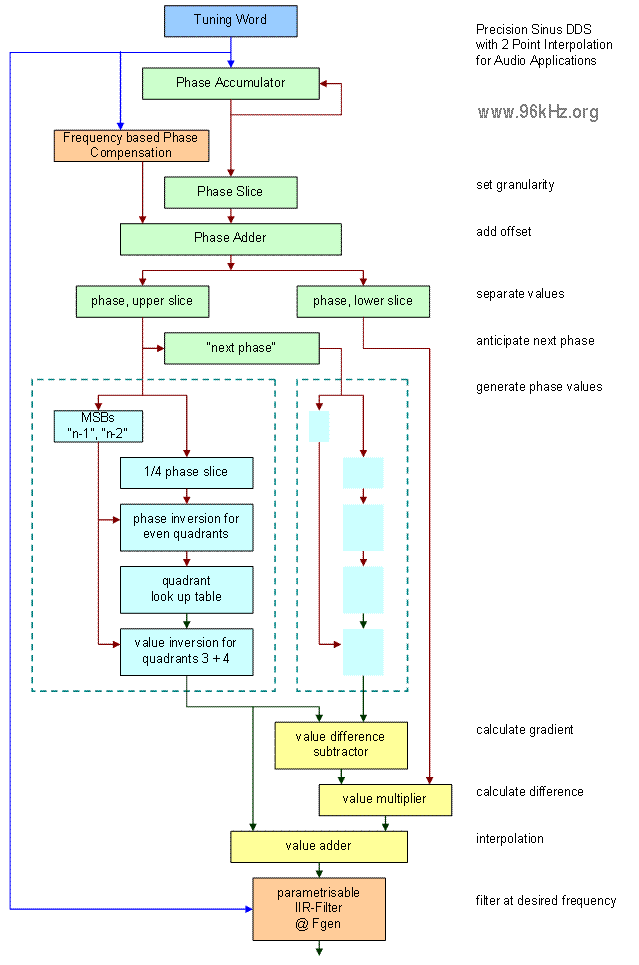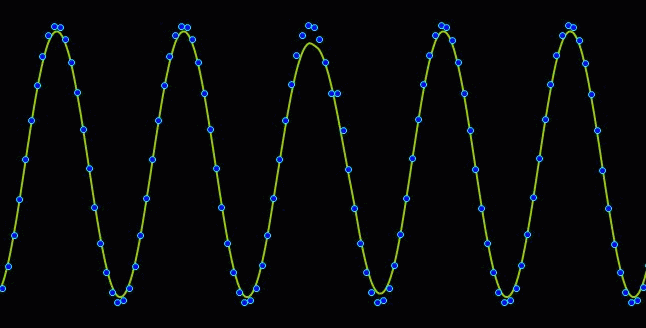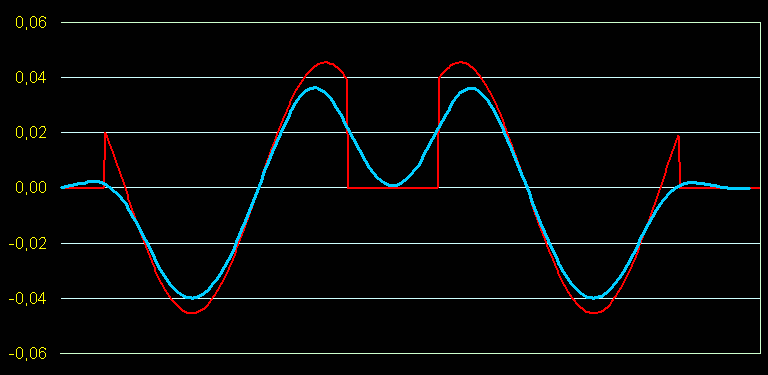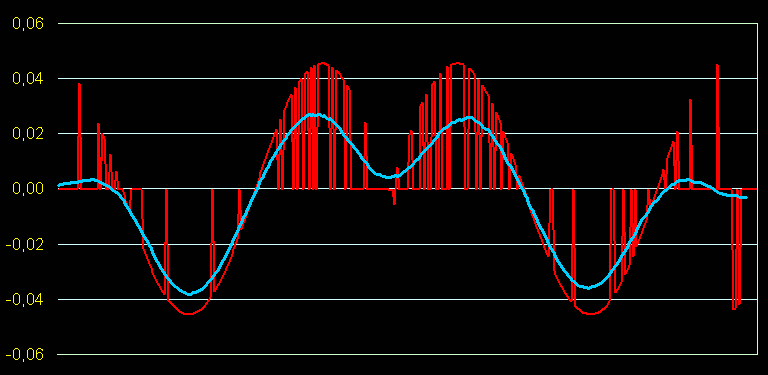96 kHz.org Advanced Audio Recording sine wave generation in digital systems For sound generation in music applications, often a precise sine wave is required. The best known method to do this directly in the digital domain is to use an approximation of the sine wave and continuously repeat it - performed by the so called DDS:   Function of DDSThe sine wave is stored in a table and read with an address counter derived from an accumulator which represents the phase. These tables can easily be generated with Excel. 90 degrees of the wave are stored and the values for other angles are than derived by angle mapping and point inversion. In the above example, interpolation is used by simultaneously calculation two points for one point of time and using the debris after cutting the phase vector (in order to obtain the address) as a parameter for a linear equation.   The limit of the phase vector Because of the fact that even with interpolation the phase vector is limited in size and also the frequency does no 100% fit into the length of the table, some points are missed or repeated during continuous stepping though the table leading to a shifted part of the sine wave and thus to a false representation. The phase errors are later filtered by the anti aliasing filter, but cannot be eliminated perfectly.Comparing the resulting value with the theoretical ideal value shows a difference which is again a sine curve over a given period. The following image gives an example:The red curve shows the absolute difference between sin(x)  and  sin(INT (k * x) /k)  representing the rounding of the phase vector. The effect is optically quite strong. The resulting wave introduces noise into the sound which is commonly filtered by the anti aliasing filter at the output leading to the cyan colored curve.The problem can be lowered using phase dithering methods. This introduces much noise at the first sight, but leads to a lower false signal after filtering because the noise has a very high frequency.   Design Recommendation To get a suitable sine wave for audio applications the DDS frequency should be at least a 100 times larger, than the highest frequency to be reproduced. For standard sound systems with 96kHz, this means that frequencies around 1kHz can be reproduced fine enough. For higher frequencies artifacts have to be accepted. This issue especially refers to approaches with non sine waves to be stored in the wave table.   See also: © 2001 J.S.